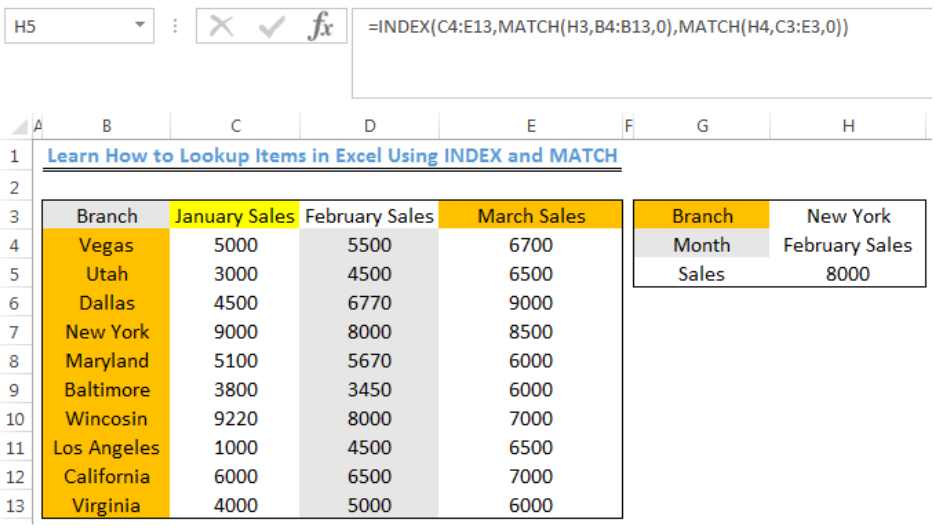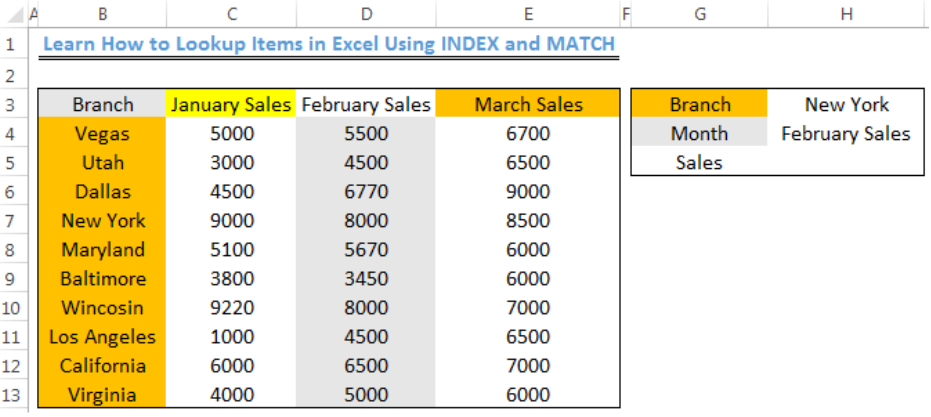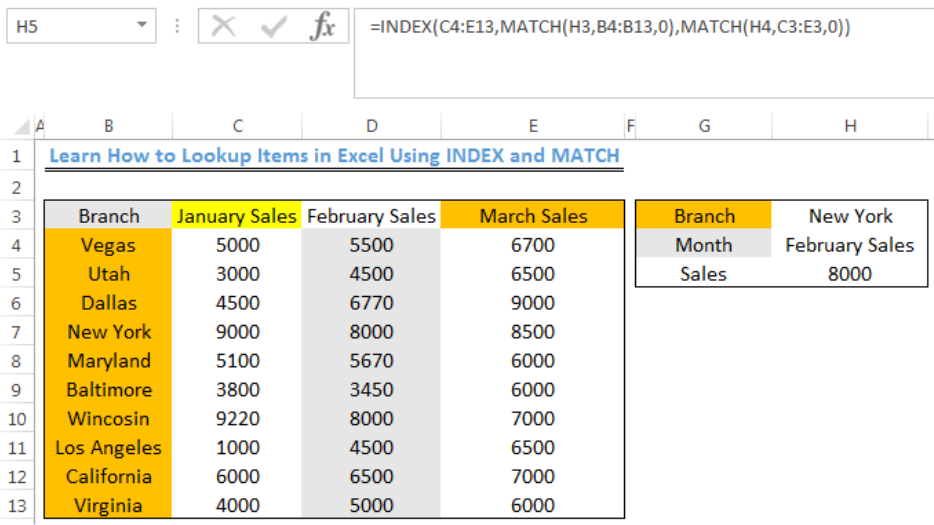Get instant live expert help with Excel or Google Sheets“My Excelchat expert helped me in less than 20 minutes, saving me what would have been 5 hours of work!”

#### Post your problem and you’ll get expert help in seconds.

Your message must be at least 40 characters
Our professional experts are available now. Your privacy is guaranteed.

# Learn How to Lookup Items in Excel Using INDEX and MATCH

Apart from VLOOKUP, we can lookup items in excel using a combined formula of INDEX and MATCH functions. This is more popular than VLOOKUP. MATCH FUNCTION returns the numeric position of an item in a list. INDEX FUNCTION returns the VALUE at a known position. The steps below will walk through the process.Figure 1- Lookup with INDEX and MATCH functions

## Formula

`=INDEX(C4:E13,MATCH(H3,B4:B13,0),MATCH(H4,C3:E3,0))`

## Setting up the Data

We will use the INDEX and MATCH functions to get the February Sales for New York Branch of a Pharmacy Store with Branches across the US

• The Branches of the store will be entered into Column B
• Column C, D, and E will contain the Sales for 3 consecutive months
• The result will be returned in Cell H5Figure 2 – Setting up the Data

## Lookup New York February Sales

• We will click on Cell H5
• We will insert the formula below into Cell H5
`=INDEX(C4:E13,MATCH(H3,B4:B13,0),MATCH(H4,C3:E3,0))`
• We will press the enter keyFigure 3- New York February Sales with the INDEX and MATCH Functions

## Explanation

• `=INDEX(C4:E13,MATCH(H3,B4:B13,0),MATCH(H4,C3:E3,0))`
• `=INDEX(array,row_num,column_num)`

• Row number: `Match(lookup_value,lookup_array,match_type)`

• Column Number: `(Match(lookup_value,lookup_array,match_type))`

In this formula, the INDEX FUNCTION is used to return the value within an array at the specified row number and column number by the MATCH FUNCTION. The returned row to INDEX is 4 and the column is 2.

`=INDEX(C4:E13,4,2)`

The INDEX function will check the range (C4:E13) and return the value in row 4 and column 2.

The first MATCH function gets the Row number of New York in the range (B4:B13) and returns as 4. The second MATCH function gets the Column number for the Month (February Sales) in the range (C3:E3) and returns as 2. This is then returned to INDEX which delivers the result.

0 in the formula means that we want an exact matching value.

## Instant Connection to an Expert through our Excelchat Service

Most of the time, the problem you will need to solve will be more complex than a simple application of a formula or function. If you want to save hours of research and frustration, try our live Excelchat service! Our Excel Experts are available 24/7 to answer any Excel question you may have. We guarantee a connection within 30 seconds and a customized solution within 20 minutes.

Solution examplesindex and match with duplicates. I need to use another column as a reference, so my return value has two match the value of two things for it to return
Solved by E. H. in 60 minsI have a file with two sheets. On the 1st, I have dollars in O13and in O16 a number signifying 1-6 people. On the second workbook is a matrix that corresponds to the dollar amount AND the number of people. For example, sheet 1 O13 is \$6,000. O16 is 3. I want a formula to find \$6,000 (not higher) on sheet 2 in column A. Then I want it to compare sheet 1 O16 to the correct column (B-G representing 1-6) on sheet 2 for an exact match to place in sheet1 O17. The range of sheet1 O13 is referenced on sheet2 A5-A383, and sheet 1 O16 is referenced on sheet2 B5:G383. I have an INDEX Match but it isn't quite working, yet.
Solved by B. B. in 60 minsI have two lists. One is a list of part numbers. The other is a list of long text descriptions. I can use VLOOKUP with "*"&cell address"*" to find the first match. But how can I find the second and third matches? I have tried using INDEX and SHORT but the wild cards no longer find the match like they do in VLOOKUP. Can you help?
Solved by S. J. in 36 minsI need a formula that will compare multiple columns, and return the column header name of whichever column had the highest value. I am using (INDEX(\$F\$1:\$K\$1,0,MATCH(MAX(F2:K2),F2:K2,0))) , but one row had all the same values and the formula returned the first column header as being the "winner". This formula cannot distinguish when there are ties.
Solved by M. C. in 51 minsHello, I have a big spreadsheet in which I need to know how many patients came in each month based on provider. I am using the following formula but I still get the N/A error. =INDEX('No Show Appts Data'!D:E, MATCH(1, 'No Show Appts Data'!D:D='No Shows Data'!M2)*('No Shows Data'!E:E='No Shows Data'!N1),0)) No Show Appts Data is the name of the sheet where Column D is Month Year of date in question and Column E is the provider. No Shows Data is the sheet where I am making all the formula calculation where Column M is Month and Year and Column N is the provider in question and therefore M2 is the month in question and N1 the provider in question. How do I fix this error? Thanks
Solved by F. H. in 40 mins